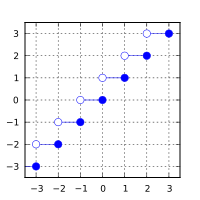/C++

# std::ceil, std::ceilf, std::ceill

Defined in header `<cmath>`
(1)
`float       ceil ( float arg );`
`float       ceilf( float arg );`
(since C++11)
`double      ceil ( double arg );`
(2)
(3)
`long double ceil ( long double arg );`
`long double ceill( long double arg );`
(since C++11)
`double      ceil ( IntegralType arg );`
(4) (since C++11)
1-3) Computes the smallest integer value not less than `arg`.
4) A set of overloads or a function template accepting an argument of any integral type. Equivalent to 2) (the argument is cast to `double`).

### Parameters

 arg - floating point value

### Return value

If no errors occur, the smallest integer value not less than `arg`, that is ⌈arg⌉, is returned.

Return valueArgument

### Error handling

Errors are reported as specified in `math_errhandling`.

If the implementation supports IEEE floating-point arithmetic (IEC 60559),

• The current rounding mode has no effect.
• If `arg` is ±∞, it is returned unmodified
• If `arg` is ±0, it is returned, unmodified
• If arg is NaN, NaN is returned

`FE_INEXACT` may be (but isn't required to be) raised when rounding a non-integer finite value.

The largest representable floating-point values are exact integers in all standard floating-point formats, so this function never overflows on its own; however the result may overflow any integer type (including `std::intmax_t`), when stored in an integer variable.

This function (for double argument) behaves as if (except for the freedom to not raise `FE_INEXACT`) implemented by the following code:

```#include <cmath>
#include <cfenv>
#pragma STDC FENV_ACCESS ON
double ceil(double x)
{
double result;
int save_round = std::fegetround();
std::fesetround(FE_UPWARD);
result = std::rint(x); // or std::nearbyint
std::fesetround(save_round);
return result;
}```

### Example

```#include <cmath>
#include <iostream>
int main()
{
std::cout << std::fixed
<< "ceil(+2.4) = " << std::ceil(+2.4) << '\n'
<< "ceil(-2.4) = " << std::ceil(-2.4) << '\n'
<< "ceil(-0.0) = " << std::ceil(-0.0) << '\n'
<< "ceil(-Inf) = " << std::ceil(-INFINITY) << '\n';
}```

Output:

```ceil(+2.4) = 3.000000
ceil(-2.4) = -2.000000
ceil(-0.0) = -0.000000
ceil(-Inf) = -INF```